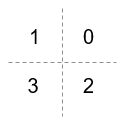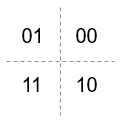# comm.QPSKModulator

(To be removed) Modulate using QPSK method

comm.QPSKModulator will be removed in a future release. Use `pskmod` instead. For information on updating your code, see Version History.

## Description

The `comm.QPSKModulator` object modulates a signal using the quadrature phase shift keying (QPSK) method. The output is a baseband representation of the modulated signal.

To modulate using the QPSK method:

1. Create the `comm.QPSKModulator` object and set its properties.

2. Call the object with arguments, as if it were a function.

## Creation

### Syntax

``qpskmod = comm.QPSKModulator``
``qpskmod = comm.QPSKModulator(Name,Value)``
``qpskmod = comm.QPSKModulator(phase,Name,Value)``

### Description

``qpskmod = comm.QPSKModulator` creates a System object™ to modulate input signals using the QPSK method.`

example

````qpskmod = comm.QPSKModulator(Name,Value)` sets properties using one or more name-value arguments. For example, `'OutputDataType'='single'` specifies output of the modulated signal values in single precision data type.```
````qpskmod = comm.QPSKModulator(phase,Name,Value)` sets the `PhaseOffset` property to `phase` and optional name-value arguments. Specify `phase` in radians.```

## Properties

expand all

Unless otherwise indicated, properties are nontunable, which means you cannot change their values after calling the object. Objects lock when you call them, and the `release` function unlocks them.

If a property is tunable, you can change its value at any time.

Phase of zeroth point of the signal constellation in radians, specified as a scalar.

Example: `'PhaseOffset',0` aligns the QPSK signal constellation points on the axes, {(1,0), (0,j), (-1,0), (0,-j)}.

Data Types: `double`

Option to provide input in bits, specified as a logical `0` (`false`) or `1` (`true`).

• When this property is set to `false`, the input values must be integer representations of two-bit input segments in the range [0, 3].

• When this property is set to `true`, the input must be a binary vector of even length. Element pairs are binary representations of integers.

Data Types: `logical`

Symbol encoding mapping of constellation bits, specified as `'Gray'` or `'Binary'`.

SettingConstellation Mapping for IntegersConstellation Mapping for BitsComment

`Gray`Map symbols using Gray-coded ordering.

`Binary`Map symbols using natural binary-coded ordering. The signal constellation maps to the complex value ```ej(PhaseOffset + (2πm/4))```, where `m` is an integer in the range [0, 3].

Data Types: `char`

Data type assigned to output, specified as `'double'`, `'single'`, or `'Custom'`.

Data Types: `char`

Fixed-Point Properties

Fixed-point data type of output, specified as a `numerictype` (Fixed-Point Designer) object with a signedness of Auto.

#### Dependencies

This property applies when you set the `OutputDataType` property to `'Custom'`.

## Usage

### Syntax

``y = qpskmod(x)``

### Description

````y = qpskmod(x)` returns baseband-modulated output.```

### Input Arguments

expand all

Input signal, specified as an NS-element column vector of integers or bits, where NS is the number of samples.

The setting of the BitInput property determines the interpretation of the input vector.

Data Types: `double` | `int8` | `logical` | `fi`

### Output Arguments

expand all

Output QPSK-modulated signal, returned as a complex-valued vector.

## Object Functions

To use an object function, specify the System object as the first input argument. For example, to release system resources of a System object named `obj`, use this syntax:

`release(obj)`

expand all

 `constellation` Calculate or plot ideal signal constellation
 `step` Run System object algorithm `release` Release resources and allow changes to System object property values and input characteristics `reset` Reset internal states of System object

## Examples

collapse all

Estimate the bit error rate of a QPSK modulated signal filtered through an AWGN channel.

Create a QPSK modulator and demodulator pair that operate on bits.

```qpskModulator = comm.QPSKModulator('BitInput',true); qpskDemodulator = comm.QPSKDemodulator('BitOutput',true);```

Create an AWGN channel object and an error rate counter.

```channel = comm.AWGNChannel('EbNo',4,'BitsPerSymbol',2); errorRate = comm.ErrorRate;```

Generate random binary data and apply QPSK modulation.

```data = randi([0 1],1000,1); txSig = qpskModulator(data);```

Pass the signal through the AWGN channel and demodulate it.

```rxSig = channel(txSig); rxData = qpskDemodulator(rxSig);```

Calculate the error statistics. Display the BER.

```errorStats = errorRate(data,rxData); errorStats(1)```
```ans = 0.0100 ```

## Version History

Introduced in R2012a

collapse all

### R2023a: To be removed

`comm.QPSKModulator` will be removed in a future release. Use the `pskmod` function with the modulation order set to `4` to QPSK modulate the input signal.